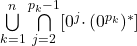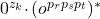# Monthly Archives: February 2021

## Regular Expression Inequivalence on Single Character Alphabets

I wanted to pause here to do a variant on the old Regular Expression Inequivalence problem that I think is both interesting and also will be used coming up.

The problem: Regular Expression Inequivalence on Single Character Alphabets.  This problem isn’t in the appendix, though it is mentioned as a comment to the normal Regular Expression Inequivalence problem (AL9).

The description: Given two regular expressions E1 and E2 that use the operators {∪, ⋅, *} and the alphabet ∑, where |∑| = 1.  Do E1 and E2 represent different languages?

Example: Suppose E1 = (aa)* ∪ (aaa)* and E2 = aa(a)*.  These languages both generate all strings of 2 or more a’s, and so are equivalent (and so would be a “no” instance of our problem).  If we changed E2 to a(a)*, then E2 could generate the string “a”, and E1 can’t, meaning the languages are inequivalent (and so would be a “yes” instance of our problem).

Reduction: Stockmeyer and Meyer talk about this problem, reducing from 3SAT.

So we’re given a formula A in CNF form, with m clauses and n variables.  The set of literals in clause k is Ck.  Now list out the first n primes p1 through pn.

We are going to consider integers z who have the value 0 or 1 mod pi for each pi.  So, for example, if we had 4 variables, the first 4 primes are 2,3,5, and 7.  15 is 1 mod 2, 0 mod 3, 0 mod 5, and 1 mod 7.  We’d represent 15 as the string “1001” and interpret it as setting variables 1 and 4 to true, and variables 2 and 3 to false.  The integer z “satisfies” A if the truth values assigned above satisfy A.

Now let’s build an expression E0 whose language is 0z for all z’s that do not generate legal encodings (they have a value that is not 0 or 1 mod some pi).  They say that expression is:So basically for each pk and each possible remainder j from 2 on up, create a string whose size is j mod pk.

Now, for each clause Ck we make an expression Ek that accepts strings 0z, where if z is a legal encoding (it’s 0 or 1 mod all pk), then it does not set any of the literals in clause k to true (meaning it is a 0 in the position of a variable that exists positively, and a 1 in the position of a variable that exists negatively).  The expression for this is:.

..where r, s, and t are the variables that occur in Ck.

Our actual first expression E1 unions E0 and all of the Ek‘s together.  E2 will be 0*.

They then say that “it is not hard to show” that A is satisfiable if and only if there is an integer z that encodes an assignment to A and 0z is not in the language of E2.  Which I think makes sense because if A was satisfiable, then the z of that assignment would not be in E0 (because it is 0 or 1 mod all pk) and it would not be in any Ek (because by satisfying clause k, the z would have to make one of the variables true).

Difficulty: 8.  I know I rated the original “Regular Expression Inequivalence” problem a 9, but that was one of the first problems I did, and since then I’ve seen some things.  I’d probably rate it a 7 now.  This version is harder, because of all the prime number issues involved.  I do think it’s possible to see how and why each expression is made, and what it’s used for, and it all comes together in a neat way.

## Covering for Linear Grammars

Here’s another covering problem, similar to the last one.

The problem: Covering for linear grammars.  This is problem AL12 in the appendix.

The description: Given two linear context-free grammars G1 = (N1, Σ, Π1, S1) and G2 = (N2, Σ, Π2, S2), where all productions have at most one nonterminal symbol on the right-hand side.  Can we create a function h where:

1. h maps productions from Π1 to productions in Π2
2. h also maps sequences of productions from ∏1* to sequences of productions in ∏2* (or the empty production λ), by defining:
1. h(ε) = ε (the empty string)
2. h(πp) = h(π)h(p) for all π in Π1* and p in Π1
3. If we can get some string w from start symbol S1 in G1 following a sequence of productions p1p2..pn, we can get w from S2 in G2 by following the sequence h(p1)h(p2)..h(pn).
4. If we can get some string w from start symbol S2 in G2 by following a sequence of productions q1q2..qn, then there is some sequence of productions p1p2..pm in G1 that also make w, and where h(p1)h(p2)..h(pm) also generate w.

This definition is actually from the paper by Hunt, Rosenkrantz, and Szymanski that has the reduction.  G&J talk about “P” in their definition after talking about “Π” in the grammar definition for the production.  I can’t tell if P is a typo for Π, or they left out the definition of P as a sequence of productions from Π.  I think the second is more likely since it fits closer to the definition above.

Example: It’s actually pretty hard to come up with an example that is not a trivial substitution.  Here are two linear grammars for “strings over {a,b} that end in a”

G1 is:

1. S->aTa
2. S->bTa
3. S->a
4. T->aT
5. T->bT
6. T->ε

G2 is:

1. S->aS
2. S->bS
3. S->a

Our function will be:

• h(S->aTa) = S->aS
• h(S->bTa) = S->bS
• h(S->a) = S->a
• h(T->aT) = S->aS
• h(T->bT) = S->bS
• h(T->ε) = S->a

So, the way we get the string “aba” in G1 is:

• S->aTa (production 1)
• ->abTa (production 5)
• ->aba (production 6)

This would become replaced by:

• S->aS (production 1 in G2)
• ->abS (production 2 in G2)
• ->aba (production 3 in G2)

Notice that in general the existence of a covering between G1 and G2 means that they have the same language.  (This is presented as an “obvious fact” without proof in the paper)

Reduction: Hunt, Rosenkrantz, and Szymanski show this is true for all regular grammars, not just linear grammars, and go from Regular Expression Non-Universality.  (Which is really just “Regular Expression Inequivalence” where one of our expressions is {0,1}*)

So, we’re given a linear grammar that can represent any regular expression.  It has a set N of nonterminals and S1 as its start symbol.  We build our own G2 with start symbol S, with productions as follows:

• S->0S
• S->1S
• S->0
• S->1

Now consider a function h that maps any production of the form A-> α, where A ∈ N, and α is whatever the result of the production in G1 was.

So h(A->α) =

• S->oS if α = 0N, where N is some non-terminal in G1
• S->1S if α = 1N
• S->0 if α = 0
• S->1 if α = 1

(Notice that these are the only possibilities if G1 was regular over {0,1})

The paper claims that G1 covers G2 under h if and only if G1‘s language was {0,1}*

Suppose G1‘s language wasn’t {0,1}*.  Well, G2‘s language sure is, so no covering can’t exist, since the languages are different.

Supposed G1‘s language was {0,1}*.  Then you can show by induction on the length of a derivation that if S1 =>* wA under some sequence of productions π that S =>* wS under the set of productions h(π), and thus S1=>* w under the sequence of productions π implies that S =>* w under the sequence of productions h(π).

We need to show the other direction of the covering definiti0n.  Suppose we have S =>* w following some set of productions π in G2. Since we’re assuming G1‘s language was {0,1}*, there has to be some sequence of productions π’ where S1=>w following π’.  We just showed that if that’s the case, then S=>* w following h(π’).  G was built to be unambiguous, so it must be the case that h(π’) = π.  This gets us what we need.

So, this h works in all cases that an h can work (if G1 wasn’t {0,1}*, no covering is possible).

Difficulty: 8, for the reduction.  I’d be a little worried about teaching this in a class because this reduction is from regular expression universality, not regular expression non-universality, in the sense that a “yes” to this problem happens in the situations where the grammar we’re given is {0,1}* (a “no” to the no-universality question).  It works for this problem in the paper because they’re actually showing that this problem is PSPACE-Complete, and PSPACE is closed under complement.  But unless I’m doing a whole class in complexity theory, I don’t think that I’d want to explain all of that.

Additionally, this is a very hard problem to explain, and I’m not 100% sure I explained it all correctly.